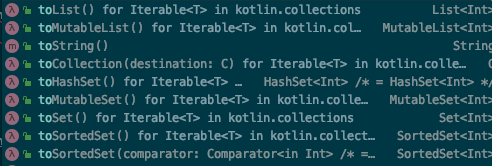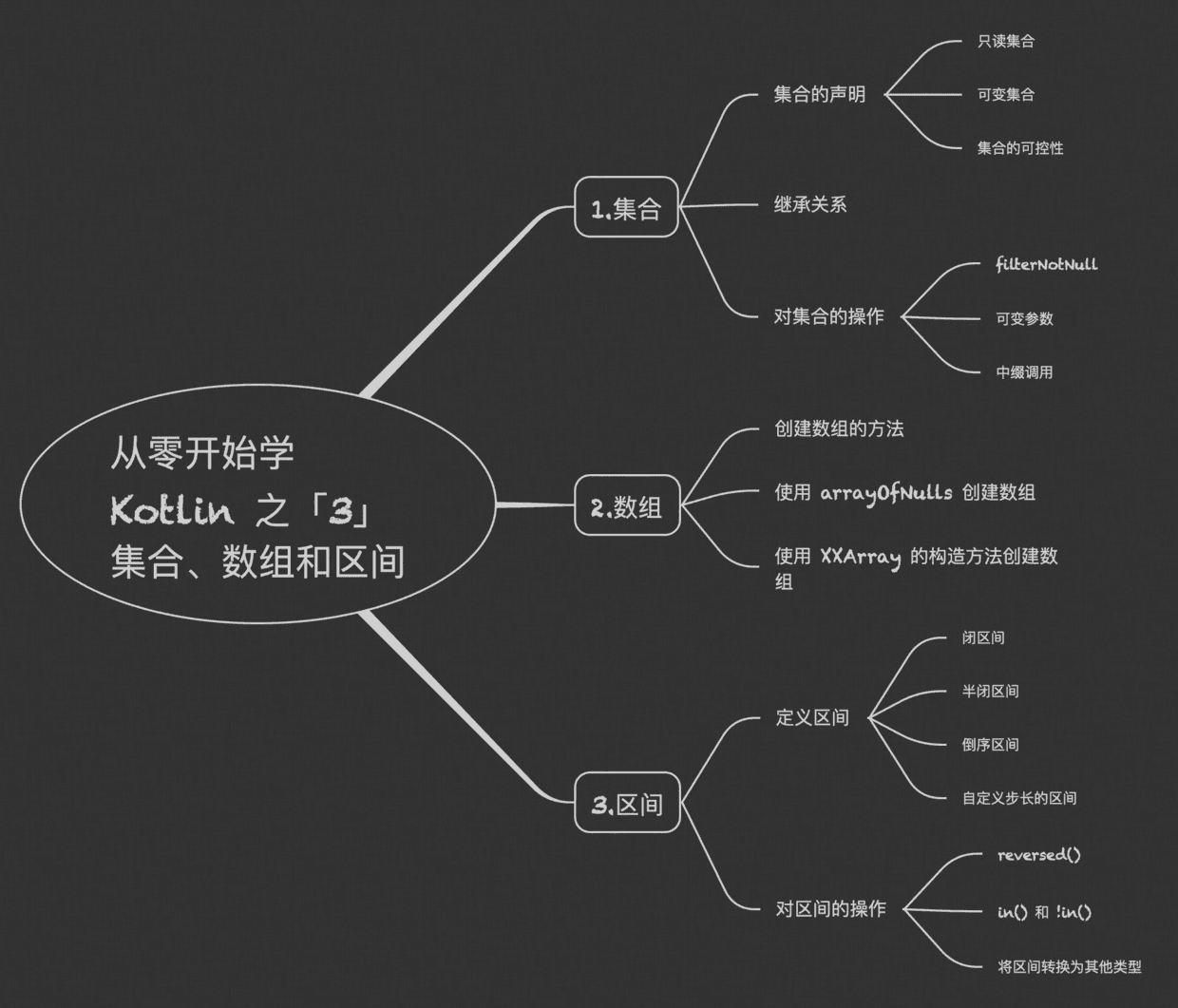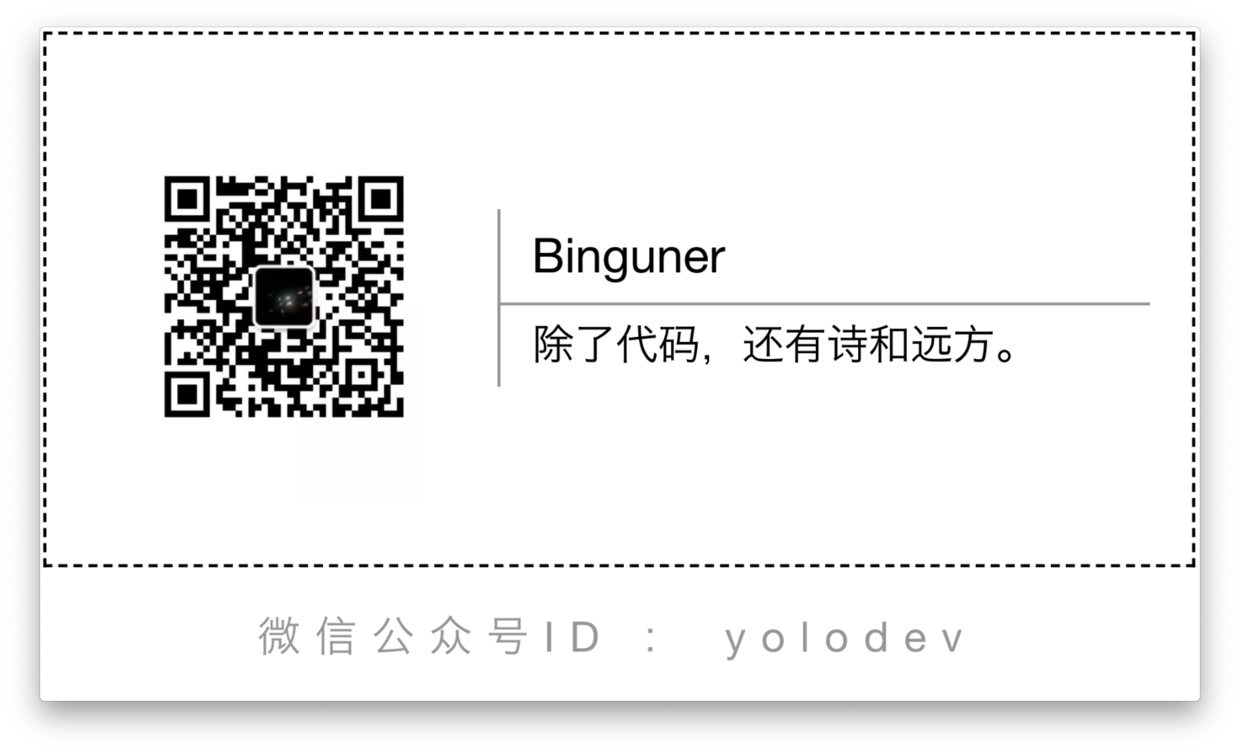# 01 只读集合 和 可变集合

Kotlin 中的集合和 Java 中的集合不一样，分为只读集合可变集合

#### 01 - 1 集合的声明

• 只读集合

val numlist = listOf(1,2,3)
val numArraylist = arrayListOf(4,5,6)
val numHashSet = hashSetOf(7,8,9)
val numHashMap = hashMapOf("a" to 10,"b" to 11,"c" to 12)

println(numlist)  // 输出 [1, 2, 3]
println(numArraylist)  //输出[4, 5, 6]
println(numHashSet)  //输出[4, 5, 6]
println(numHashMap)  //输出{a=10, b=11, c=12}


• 可变集合

val numMutablelist = mutableListOf(1,2,3)
val numMutableHashSet = mutableSetOf(7,8,9)
val numMutableHashMap = mutableMapOf("a" to 10,"b" to 11,"c" to 12)

println(numMutablelist)  // 输出[1, 2, 3]
println(numMutableHashSet)  // 输出[7, 8, 9]
println(numMutableHashMap)  // 输出{a=10, b=11, c=12}

println(numMutablelist)  // 输出[1, 2, 3, 4]

• 集合的可空性

Kotlin 支持类型参数的可空性。
Kotlin 中所有的泛型和泛型函数的类型参数都是可空的。

var list = mutableListOf<Int?>()


#### 01 - 3 对集合的操作

1. filterNotNull()

var list = listOf(1,2,3,null,4,null);
println(list)  // 输出 [1, 2, 3, null, 4, null]
println(list.filterNotNull())  // 输出 [1, 2, 3, 4]

1. 可变参数

Kotlin 中使用 vararg 来声明一个可变的参数。查看 listOf 的源码，就可以看到 vararg 的使用。

public fun <T> listOf(vararg elements: T): List<T> = if (elements.size > 0) elements.asList() else emptyList()

1. 中缀调用（infix function）

kotlin 中的 mapOf 函数就使用了中缀调用。

var map = mapOf(1 to "one",2 to "two", 3 to "three")


public infix fun <A, B> A.to(that: B): Pair<A, B> = Pair(this, that)


Pair 类型表示一对元素

# 02 数组

#### 02 - 1 创建数组

Kotlin 为了使用数组，增加了 ArrayArrays

• Array 由 arrayOf() 创建并返回，可以生成任意参数类型的数组。
• Arrays 可以创建各种基本数据类型的数组，比如说 ByteArray、CharArray、ShortArray、IntArray、LongArray、FloatArray、DoubleArray、BooleanArray，对应的生成方法是 byteArrayOf()、charArrayOf() 等。

var strArray = arrayOf("2","a","3")
println(strArray.joinToString(" "))  // 输出 2 a 3

var intArray = intArrayOf(1,2,3,4,5,3)
println(intArray.joinToString(" "))  // 输出 1 2 3 4 5 3


#### 02 - 2 其他创建数组的方法

1. 使用 arrayOfNulls

var array = arrayOfNulls<Int>(3)
array = 3

println(array.joinToString(" "))  // 输出 null null 3

1. 使用 Array 的构造方法

var array = IntArray(4){
i -> i * 2
}
var array2 = BooleanArray(3)

println(array.joinToString(" "))  // 输出 0 2 4 6
println(array2.joinToString(" "))  // 输出 false false false


# 03 区间

Kotlin 中区间的概念和数学上的差不多，也用来表示一个范围，它是由具有操作符形式 ..rangeTo 函数定义的。

#### 03 - 1 定义区间

• 闭区间
var range = 1 .. 5

for ( i in range){
print("$i ") } // 输出 1 2 3 4 5  • 半闭区间 var range = 1 until 5 for ( i in range){ print("$i ")  // 输出 1 2 3 4
}

• 倒序区间
var range = 5 downTo 1
for ( i in range){
print("$i ") } // 输出 5 4 3 2 1  • 自定义步长的区间 var range = 1..5 step 2 for (i in range){ print("$i ")  // 输出 1 3 5
}

• 反转序列
// 先定义一个 1 到 5 到区间
var range = 1 .. 5
// 对这个区间调用 reversed()
var range2 = range.reversed()

for ( i in range2){
print("\$i ")  // 输出 5 4 3 2 1
}


#### 03 - 2 对区间的操作

###### 1. in 和 !in

in 用来检查某个对象是否属于集合，它对应对函数是 contains
!in 用来检查某个对象是否不属于集合 。

var range = 1 .. 5
println( 1 in range)  // 输出 true
println( 1 !in range1)  // 输出 false

###### 2. 将区间转换为其他类型

Kotlin 提供了一下方法可以直接将区间转换为集合

var range1 = 1 .. 5
var list = range1.toList()
println(list)  // 输出 [1, 2, 3, 4, 5]# 最后

「从零开始学 Kotlin 之『3』集合、数组和区间」到这里就结束了。©️2019 CSDN 皮肤主题: 大白 设计师: CSDN官方博客